### One Nation Under Bush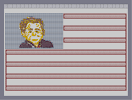Hover over the thumbnail for a full-size version.

Author toby3p0 author:toby3p0 n-art rated 2006-11-27 4 by 12 people. \$One Nation Under Bush#toby3p0#none#00000000000000000000000000000000000000000000000000000000000000000000000000000000000000000000000000000000000000000000000000000000000000000000000000000000000000000000000000000000000000000000000000000000000000000000000000000000000000000000000000000000000000000000000000000000000000000000000000000000000000000000000000000000000000000000000000000000000000000000000000000000000000000000000000000000000000000000000000000000000000000000000000000000000000000000000000000000000000000000000000000000000000000000000000000000000000000000000000000000000000000000000000000000000000000000000000000000000000000000000000000000000000000000000000000000000000000000000000000000000000000000000000000000000000000000000000000000000000000|12^756,84!12^756,96!12^756,108!12^756,156!12^756,168!12^756,180!12^756,228!12^756,240!12^756,252!12^756,300!12^756,312!12^756,324!12^756,372!12^756,384!12^756,396!12^756,444!12^756,456!12^756,468!12^756,516!12^756,528!12^756,540!12^36,300!12^36,312!12^36,324!12^36,372!12^36,384!12^36,396!12^36,444!12^36,456!12^36,468!12^36,516!12^36,528!12^36,540!12^744,84!12^732,84!12^720,84!12^708,84!12^696,84!12^684,84!12^672,84!12^660,84!12^648,84!12^636,84!12^624,84!12^612,84!12^600,84!12^588,84!12^576,84!12^564,84!12^552,84!12^540,84!12^528,84!12^504,84!12^516,84!12^492,84!12^480,84!12^468,84!12^456,84!12^444,84!12^432,84!12^420,84!12^408,84!12^396,84!12^384,84!12^744,156!12^744,180!12^744,108!12^744,228!12^744,252!12^744,300!12^744,324!12^744,372!12^744,396!12^744,444!12^744,468!12^744,516!12^744,540!12^48,324!12^48,372!12^48,396!12^48,444!12^48,468!12^48,516!12^48,540!12^732,108!12^720,108!12^708,108!12^684,108!12^696,108!12^672,108!12^660,108!12^648,108!12^636,108!12^612,108!12^600,108!12^624,108!12^588,108!12^564,108!12^552,108!12^528,108!12^516,108!12^504,108!12^480,108!12^456,108!12^432,108!12^420,108!12^408,108!12^384,108!12^396,108!12^444,108!12^468,108!12^492,108!12^540,108!12^576,108!12^732,156!12^720,156!12^708,156!12^696,156!12^684,156!12^672,156!12^648,156!12^660,156!12^636,156!12^624,156!12^612,156!12^600,156!12^588,156!12^576,156!12^564,156!12^552,156!12^540,156!12^528,156!12^504,156!12^516,156!12^492,156!12^480,156!12^468,156!12^456,156!12^444,156!12^432,156!12^420,156!12^408,156!12^396,156!12^384,156!12^732,180!12^720,180!12^708,180!12^696,180!12^684,180!12^672,180!12^660,180!12^648,180!12^636,180!12^624,180!12^612,180!12^600,180!12^588,180!12^576,180!12^564,180!12^552,180!12^540,180!12^528,180!12^516,180!12^504,180!12^492,180!12^480,180!12^468,180!12^456,180!12^444,180!12^432,180!12^420,180!12^408,180!12^396,180!12^384,180!12^732,228!12^720,228!12^708,228!12^684,228!12^696,228!12^672,228!12^660,228!12^648,228!12^636,228!12^624,228!12^612,228!12^600,228!12^588,228!12^564,228!12^576,228!12^552,228!12^540,228!12^528,228!12^516,228!12^504,228!12^480,228!12^492,228!12^456,228!12^468,228!12^444,228!12^420,228!12^432,228!12^408,228!12^396,228!12^384,228!12^732,252!12^720,252!12^708,252!12^696,252!12^684,252!12^672,252!12^660,252!12^648,252!12^636,252!12^624,252!12^600,252!12^612,252!12^588,252!12^576,252!12^564,252!12^540,252!12^552,252!12^528,252!12^516,252!12^504,252!12^492,252!12^480,252!12^468,252!12^456,252!12^444,252!12^432,252!12^420,252!12^408,252!12^396,252!12^384,252!12^732,300!12^720,300!12^708,300!12^696,300!12^684,300!12^672,300!12^660,300!12^648,300!12^636,300!12^624,300!12^612,300!12^600,300!12^588,300!12^576,300!12^564,300!12^552,300!12^540,300!12^528,300!12^516,300!12^504,300!12^492,300!12^480,300!12^468,300!12^456,300!12^444,300!12^432,300!12^420,300!12^408,300!12^396,300!12^384,300!12^372,300!12^360,300!12^348,300!12^336,300!12^324,300!12^312,300!12^300,300!12^288,300!12^276,300!12^264,300!12^240,300!12^252,300!12^228,300!12^216,300!12^204,300!12^192,300!12^180,300!12^168,300!12^156,300!12^144,300!12^132,300!12^120,300!12^108,300!12^96,300!12^84,300!12^72,300!12^60,300!12^48,300!12^60,324!12^72,324!12^84,324!12^96,324!12^108,324!12^120,324!12^132,324!12^144,324!12^156,324!12^168,324!12^180,324!12^192,324!12^216,324!12^204,324!12^228,324!12^240,324!12^252,324!12^264,324!12^276,324!12^288,324!12^300,324!12^312,324!12^324,324!12^348,324!12^336,324!12^360,324!12^372,324!12^384,324!12^396,324!12^408,324!12^408,324!12^420,324!12^432,324!12^444,324!12^456,324!12^468,324!12^480,324!12^492,324!12^504,324!12^516,324!12^528,324!12^540,324!12^552,324!12^552,324!12^564,324!12^588,324!12^576,324!12^600,324!12^612,324!12^624,324!12^636,324!12^648,324!12^660,324!12^672,324!12^684,324!12^696,324!12^708,324!12^720,324!12^732,324!12^732,372!12^720,372!12^708,372!12^684,372!12^696,372!12^672,372!12^672,372!12^660,372!12^648,372!12^636,372!12^624,372!12^612,372!12^600,372!12^588,372!12^576,372!12^564,372!12^552,372!12^552,372!12^540,372!12^528,372!12^516,372!12^504,372!12^492,372!12^480,372!12^468,372!12^456,372!12^444,372!12^432,372!12^420,372!12^408,372!12^396,372!12^384,372!12^372,372!12^360,372!12^336,372!12^348,372!12^324,372!12^312,372!12^300,372!12^288,372!12^276,372!12^264,372!12^252,372!12^240,372!12^228,372!12^216,372!12^204,372!12^192,372!12^180,372!12^168,372!12^156,372!12^144,372!12^132,372!12^120,372!12^108,372!12^96,372!12^96,372!12^84,372!12^72,372!12^60,372!12^60,396!12^72,396!12^84,396!12^96,396!12^108,396!12^120,396!12^132,396!12^144,396!12^156,396!12^168,396!12^180,396!12^192,396!12^204,396!12^216,396!12^228,396!12^240,396!12^252,396!12^276,396!12^264,396!12^288,396!12^300,396!12^312,396!12^324,396!12^336,396!12^348,396!12^348,396!12^360,396!12^372,396!12^384,396!12^396,396!12^408,396!12^420,396!12^432,396!12^432,396!12^444,396!12^456,396!12^468,396!12^480,396!12^480,396!12^492,396!12^504,396!12^516,396!12^528,396!12^540,396!12^552,396!12^564,396!12^576,396!12^588,396!12^600,396!12^612,396!12^624,396!12^636,396!12^648,396!12^660,396!12^672,396!12^684,396!12^696,396!12^708,396!12^720,396!12^732,396!12^732,444!12^708,444!12^720,444!12^696,444!12^684,444!12^672,444!12^660,444!12^648,444!12^636,444!12^624,444!12^612,444!12^600,444!12^600,444!12^576,444!12^564,444!12^588,444!12^552,444!12^540,444!12^528,444!12^516,444!12^504,444!12^492,444!12^480,444!12^468,444!12^456,444!12^444,444!12^432,444!12^420,444!12^408,444!12^396,444!12^384,444!12^372,444!12^360,444!12^348,444!12^336,444!12^312,444!12^324,444!12^300,444!12^288,444!12^276,444!12^264,444!12^252,444!12^240,444!12^228,444!12^216,444!12^216,444!12^192,444!12^180,444!12^168,444!12^156,444!12^132,444!12^132,444!12^120,444!12^108,444!12^96,444!12^84,444!12^72,444!12^60,444!12^144,444!12^204,444!12^60,468!12^72,468!12^84,468!12^96,468!12^108,468!12^120,468!12^132,468!12^168,468!12^156,468!12^144,468!12^180,468!12^192,468!12^204,468!12^216,468!12^228,468!12^240,468!12^252,468!12^264,468!12^276,468!12^288,468!12^300,468!12^312,468!12^336,468!12^324,468!12^348,468!12^360,468!12^384,468!12^372,468!12^396,468!12^408,468!12^432,468!12^420,468!12^432,468!12^444,468!12^456,468!12^468,468!12^480,468!12^492,468!12^504,468!12^528,468!12^516,468!12^540,468!12^552,468!12^564,468!12^576,468!12^588,468!12^600,468!12^612,468!12^624,468!12^648,468!12^636,468!12^660,468!12^672,468!12^684,468!12^696,468!12^708,468!12^720,468!12^732,468!12^732,516!12^708,516!12^720,516!12^696,516!12^684,516!12^672,516!12^660,516!12^648,516!12^636,516!12^624,516!12^612,516!12^600,516!12^588,516!12^576,516!12^564,516!12^552,516!12^540,516!12^528,516!12^516,516!12^504,516!12^492,516!12^480,516!12^468,516!12^444,516!12^456,516!12^432,516!12^420,516!12^408,516!12^396,516!12^384,516!12^372,516!12^360,516!12^348,516!12^336,516!12^324,516!12^312,516!12^300,516!12^288,516!12^276,516!12^264,516!12^252,516!12^240,516!12^228,516!12^216,516!12^204,516!12^192,516!12^192,516!12^180,516!12^168,516!12^156,516!12^144,516!12^132,516!12^132,516!12^120,516!12^108,516!12^96,516!12^84,516!12^72,516!12^60,516!12^60,540!12^72,540!12^84,540!12^96,540!12^108,540!12^120,540!12^132,540!12^144,540!12^156,540!12^168,540!12^180,540!12^192,540!12^192,540!12^204,540!12^216,540!12^228,540!12^240,540!12^252,540!12^264,540!12^276,540!12^288,540!12^300,540!12^312,540!12^324,540!12^336,540!12^348,540!12^360,540!12^372,540!12^384,540!12^396,540!12^408,540!12^420,540!12^432,540!12^444,540!12^456,540!12^468,540!12^480,540!12^492,540!12^504,540!12^516,540!12^528,540!12^540,540!12^552,540!12^564,540!12^576,540!12^588,540!12^600,540!12^612,540!12^624,540!12^636,540!12^648,540!12^660,540!12^672,540!12^684,540!12^696,540!12^708,540!12^720,540!12^732,540!12^384,96!12^408,96!12^396,96!12^384,168!12^396,168!12^408,168!12^384,240!12^396,240!12^408,240!12^48,312!12^60,312!12^72,312!12^36,384!12^48,384!12^60,384!12^72,384!12^48,456!12^60,456!12^72,456!12^48,528!12^60,528!12^72,528!12^36,504!12^36,492!12^36,480!12^36,432!12^36,420!12^36,360!12^36,348!12^36,336!12^756,120!12^756,144!12^756,132!12^756,192!12^756,204!12^756,216!12^756,264!12^756,276!12^756,288!12^756,336!12^756,348!12^756,360!12^756,408!12^756,420!12^756,432!12^756,480!12^756,492!12^756,504!12^420,96!12^432,96!12^444,96!12^456,96!12^468,96!12^480,96!12^492,96!12^504,96!12^516,96!12^528,96!12^540,96!12^564,96!12^552,96!12^576,96!12^588,96!12^600,96!12^612,96!12^624,96!12^636,96!12^648,96!12^660,96!12^672,96!12^684,96!12^696,96!12^708,96!12^720,96!12^732,96!12^744,96!12^420,168!12^444,168!12^432,168!12^456,168!12^468,168!12^480,168!12^492,168!12^504,168!12^516,168!12^528,168!12^540,168!12^552,168!12^564,168!12^576,168!12^588,168!12^600,168!12^612,168!12^624,168!12^636,168!12^648,168!12^660,168!12^672,168!12^684,168!12^696,168!12^708,168!12^720,168!12^732,168!12^744,168!12^420,240!12^432,240!12^444,240!12^456,240!12^468,240!12^480,240!12^480,240!12^492,240!12^504,240!12^516,240!12^516,240!12^528,240!12^540,240!12^552,240!12^564,240!12^564,240!12^576,240!12^588,240!12^600,240!12^612,240!12^624,240!12^636,240!12^648,240!12^660,240!12^672,240!12^684,240!12^696,240!12^708,240!12^732,240!12^720,240!12^744,240!12^84,312!12^96,312!12^108,312!12^120,312!12^132,312!12^144,312!12^156,312!12^168,312!12^180,312!12^192,312!12^204,312!12^216,312!12^228,312!12^240,312!12^252,312!12^264,312!12^276,312!12^288,312!12^300,312!12^312,312!12^324,312!12^336,312!12^348,312!12^360,312!12^372,312!12^384,312!12^396,312!12^408,312!12^420,312!12^432,312!12^444,312!12^456,312!12^468,312!12^480,312!12^492,312!12^504,312!12^516,312!12^528,312!12^540,312!12^552,312!12^564,312!12^576,312!12^588,312!12^600,312!12^624,312!12^612,312!12^636,312!12^648,312!12^660,312!12^672,312!12^684,312!12^696,312!12^708,312!12^720,312!12^732,312!12^744,312!12^84,384!12^96,384!12^108,384!12^120,384!12^132,384!12^144,384!12^156,384!12^168,384!12^180,384!12^192,384!12^204,384!12^216,384!12^228,384!12^240,384!12^252,384!12^264,384!12^276,384!12^288,384!12^300,384!12^312,384!12^324,384!12^336,384!12^348,384!12^360,384!12^372,384!12^384,384!12^396,384!12^408,384!12^432,384!12^420,384!12^444,384!12^456,384!12^468,384!12^480,384!12^492,384!12^504,384!12^516,384!12^528,384!12^540,384!12^552,384!12^564,384!12^576,384!12^588,384!12^600,384!12^612,384!12^624,384!12^636,384!12^648,384!12^660,384!12^672,384!12^684,384!12^708,384!12^696,384!12^720,384!12^732,384!12^744,384!12^84,456!12^96,456!12^120,456!12^108,456!12^132,456!12^144,456!12^156,456!12^168,456!12^180,456!12^192,456!12^204,456!12^216,456!12^228,456!12^240,456!12^252,456!12^264,456!12^276,456!12^288,456!12^300,456!12^312,456!12^324,456!12^336,456!12^348,456!12^360,456!12^372,456!12^384,456!12^396,456!12^408,456!12^420,456!12^432,456!12^444,456!12^456,456!12^468,456!12^480,456!12^492,456!12^504,456!12^516,456!12^528,456!12^540,456!12^552,456!12^564,456!12^576,456!12^588,456!12^600,456!12^612,456!12^624,456!12^636,456!12^648,456!12^660,456!12^672,456!12^684,456!12^696,456!12^708,456!12^720,456!12^732,456!12^744,456!12^84,528!12^96,528!12^108,528!12^120,528!12^132,528!12^156,528!12^156,528!12^180,528!12^204,528!12^216,528!12^240,528!12^252,528!12^276,528!12^144,528!12^168,528!12^192,528!12^228,528!12^264,528!12^288,528!12^300,528!12^312,528!12^324,528!12^336,528!12^348,528!12^360,528!12^372,528!12^384,528!12^396,528!12^408,528!12^420,528!12^432,528!12^444,528!12^456,528!12^468,528!12^480,528!12^492,528!12^504,528!12^516,528!12^528,528!12^540,528!12^552,528!12^564,528!12^576,528!12^588,528!12^600,528!12^612,528!12^624,528!12^636,528!12^648,528!12^660,528!12^672,528!12^684,528!12^696,528!12^708,528!12^720,528!12^732,528!12^744,528!11^36,36,36,84!11^36,36,372,84!11^36,36,36,288!11^36,36,372,288!11^36,36,36,96!11^36,36,36,108!11^36,36,36,120!11^36,36,36,132!11^36,36,36,144!11^36,36,36,156!11^36,36,36,168!11^36,36,36,180!11^36,36,36,192!11^36,36,36,204!11^36,36,36,216!11^36,36,36,228!11^36,36,36,240!11^36,36,36,252!11^36,36,36,264!11^36,36,36,276!11^36,36,360,84!11^36,36,348,84!11^36,36,48,84!11^36,36,60,84!11^36,36,72,84!11^36,36,84,84!11^36,36,96,84!11^36,36,108,84!11^36,36,120,84!11^36,36,132,84!11^36,36,144,84!11^36,36,156,84!11^36,36,168,84!11^36,36,180,84!11^36,36,192,84!11^36,36,204,84!11^36,36,216,84!11^36,36,228,84!11^36,36,240,84!11^36,36,252,84!11^36,36,264,84!11^36,36,288,84!11^36,36,276,84!11^36,36,300,84!11^36,36,312,84!11^36,36,324,84!11^36,36,336,84!11^36,36,372,96!11^36,36,372,108!11^36,36,372,120!11^36,36,372,132!11^36,36,372,144!11^36,36,372,156!11^36,36,372,168!11^36,36,372,180!11^36,36,372,192!11^36,36,372,204!11^36,36,372,216!11^36,36,372,240!11^36,36,372,228!11^36,36,372,252!11^36,36,372,264!11^36,36,372,276!11^36,36,48,288!11^36,36,60,288!11^36,36,72,288!11^36,36,84,288!11^36,36,96,288!11^36,36,108,288!11^36,36,120,288!11^36,36,132,288!11^36,36,144,288!11^36,36,156,288!11^36,36,168,288!11^36,36,180,288!11^36,36,192,288!11^36,36,204,288!11^36,36,216,288!11^36,36,228,288!11^36,36,240,288!11^36,36,252,288!11^36,36,264,288!11^36,36,276,288!11^36,36,288,288!11^36,36,300,288!11^36,36,312,288!11^36,36,324,288!11^36,36,336,288!11^36,36,348,288!11^36,36,360,288!12^390,84!12^402,84!12^414,84!12^426,84!12^438,84!12^450,84!12^462,84!12^474,84!12^486,84!12^498,84!12^510,84!12^522,84!12^534,84!12^546,84!12^558,84!12^570,84!12^582,84!12^594,84!12^606,84!12^618,84!12^630,84!12^642,84!12^654,84!12^666,84!12^678,84!12^690,84!12^702,84!12^714,84!12^726,84!12^738,84!12^750,84!12^756,90!12^756,102!12^756,114!12^756,126!12^756,138!12^756,150!12^756,162!12^756,174!12^756,186!12^756,198!12^756,210!12^756,222!12^756,234!12^756,246!12^756,258!12^756,270!12^756,282!12^756,294!12^756,306!12^756,318!12^756,330!12^756,342!12^756,354!12^756,366!12^756,378!12^756,390!12^756,402!12^756,414!12^756,426!12^756,438!12^756,450!12^756,462!12^756,474!12^756,486!12^756,498!12^756,510!12^756,522!12^756,534!12^36,306!12^36,318!12^36,330!12^36,342!12^36,354!12^36,366!12^36,378!12^36,390!12^36,402!12^36,420!12^36,408!12^36,414!12^36,426!12^36,438!12^36,450!12^36,462!12^36,474!12^36,486!12^36,498!12^36,510!12^36,522!12^36,534!12^42,540!12^54,540!12^66,540!12^78,540!12^90,540!12^102,540!12^114,540!12^126,540!12^138,540!12^150,540!12^162,540!12^174,540!12^186,540!12^198,540!12^210,540!12^222,540!12^234,540!12^246,540!12^258,540!12^270,540!12^282,540!12^294,540!12^306,540!12^318,540!12^330,540!12^342,540!12^354,540!12^366,540!12^378,540!12^390,540!12^402,540!12^414,540!12^426,540!12^438,540!12^450,540!12^462,540!12^474,540!12^486,540!12^498,540!12^510,540!12^522,540!12^534,540!12^546,540!12^558,540!12^570,540!12^582,540!12^594,540!12^606,540!12^618,540!12^630,540!12^642,540!12^654,540!12^666,540!12^678,540!12^690,540!12^702,540!12^714,540!12^726,540!12^738,540!12^750,540!12^384,90!12^384,102!12^384,162!12^384,174!12^750,108!12^750,156!12^750,180!12^390,108!12^402,108!12^414,108!12^426,108!12^438,108!12^450,108!12^462,108!12^474,108!12^486,108!12^498,108!12^510,108!12^522,108!12^534,108!12^546,108!12^558,108!12^570,108!12^582,108!12^594,108!12^606,108!12^618,108!12^630,108!12^642,108!12^654,108!12^666,108!12^678,108!12^690,108!12^702,108!12^714,108!12^726,108!12^738,108!12^390,156!12^402,156!12^414,156!12^426,156!12^438,156!12^450,156!12^462,156!12^474,156!12^486,156!12^498,156!12^510,156!12^522,156!12^534,156!12^546,156!12^558,156!12^570,156!12^582,156!12^594,156!12^606,156!12^618,156!12^630,156!12^642,156!12^654,156!12^666,156!12^678,156!12^690,156!12^702,156!12^714,156!12^726,156!12^738,156!12^738,180!12^726,180!12^714,180!12^702,180!12^690,180!12^678,180!12^666,180!12^654,180!12^642,180!12^630,180!12^618,180!12^606,180!12^594,180!12^582,180!12^570,180!12^558,180!12^546,180!12^534,180!12^522,180!12^510,180!12^498,180!12^486,180!12^474,180!12^462,180!12^450,180!12^438,180!12^426,180!12^414,180!12^402,180!12^390,180!12^384,234!12^384,246!12^390,228!12^402,228!12^414,228!12^426,228!12^438,228!12^450,228!12^462,228!12^474,228!12^486,228!12^498,228!12^510,228!12^522,228!12^534,228!12^546,228!12^558,228!12^570,228!12^582,228!12^594,228!12^606,228!12^618,228!12^630,228!12^642,228!12^654,228!12^666,228!12^678,228!12^690,228!12^702,228!12^714,228!12^726,228!12^738,228!12^750,228!12^750,252!12^390,252!12^738,252!12^726,252!12^714,252!12^702,252!12^690,252!12^678,252!12^666,252!12^654,252!12^642,252!12^630,252!12^618,252!12^606,252!12^594,252!12^582,252!12^570,252!12^558,252!12^546,252!12^534,252!12^522,252!12^510,252!12^498,252!12^486,252!12^474,252!12^462,252!12^450,252!12^438,252!12^426,252!12^414,252!12^402,252!12^42,300!12^54,300!12^66,300!12^78,300!12^90,300!12^102,300!12^114,300!12^126,300!12^138,300!12^150,300!12^162,300!12^174,300!12^186,300!12^198,300!12^210,300!12^222,300!12^234,300!12^246,300!12^258,300!12^270,300!12^282,300!12^294,300!12^306,300!12^318,300!12^330,300!12^342,300!12^354,300!12^366,300!12^378,300!12^390,300!12^402,300!12^414,300!12^426,300!12^438,300!12^450,300!12^462,300!12^474,300!12^486,300!12^498,300!12^510,300!12^522,300!12^534,300!12^546,300!12^558,300!12^570,300!12^582,300!12^594,300!12^606,300!12^618,300!12^630,300!12^642,300!12^654,300!12^666,300!12^678,300!12^690,300!12^702,300!12^714,300!12^726,300!12^738,300!12^750,300!12^750,324!12^738,324!12^726,324!12^714,324!12^702,324!12^690,324!12^678,324!12^666,324!12^654,324!12^642,324!12^630,324!12^618,324!12^606,324!12^594,324!12^582,324!12^570,324!12^558,324!12^546,324!12^534,324!12^522,324!12^510,324!12^498,324!12^486,324!12^474,324!12^462,324!12^450,324!12^438,324!12^426,324!12^414,324!12^402,324!12^390,324!12^378,324!12^366,324!12^354,324!12^342,324!12^330,324!12^318,324!12^306,324!12^294,324!12^282,324!12^270,324!12^258,324!12^246,324!12^234,324!12^222,324!12^210,324!12^198,324!12^186,324!12^174,324!12^162,324!12^150,324!12^138,324!12^126,324!12^114,324!12^102,324!12^90,324!12^78,324!12^66,324!12^54,324!12^42,324!12^42,372!12^54,372!12^66,372!12^78,372!12^90,372!12^102,372!12^120,372!12^114,372!12^126,372!12^138,372!12^150,372!12^162,372!12^174,372!12^186,372!12^198,372!12^210,372!12^222,372!12^234,372!12^246,372!12^258,372!12^270,372!12^282,372!12^294,372!12^306,372!12^318,372!12^330,372!12^342,372!12^354,372!12^366,372!12^378,372!12^390,372!12^402,372!12^414,372!12^426,372!12^438,372!12^450,372!12^462,372!12^474,372!12^486,372!12^498,372!12^510,372!12^522,372!12^534,372!12^546,372!12^558,372!12^570,372!12^582,372!12^594,372!12^606,372!12^618,372!12^630,372!12^642,372!12^654,372!12^666,372!12^678,372!12^690,372!12^702,372!12^714,372!12^726,372!12^738,372!12^750,372!12^750,396!12^738,396!12^726,396!12^714,396!12^702,396!12^690,396!12^678,396!12^666,396!12^654,396!12^642,396!12^630,396!12^618,396!12^606,396!12^594,396!12^582,396!12^570,396!12^558,396!12^546,396!12^534,396!12^522,396!12^510,396!12^498,396!12^486,396!12^474,396!12^462,396!12^450,396!12^438,396!12^426,396!12^414,396!12^402,396!12^390,396!12^378,396!12^366,396!12^354,396!12^342,396!12^330,396!12^318,396!12^306,396!12^294,396!12^282,396!12^270,396!12^258,396!12^246,396!12^234,396!12^222,396!12^210,396!12^198,396!12^186,396!12^174,396!12^162,396!12^150,396!12^138,396!12^126,396!12^114,396!12^102,396!12^90,396!12^78,396!12^66,396!12^54,396!12^42,396!12^42,444!12^54,444!12^66,444!12^78,444!12^90,444!12^102,444!12^114,444!12^126,444!12^138,444!12^150,444!12^162,444!12^174,444!12^186,444!12^198,444!12^210,444!12^222,444!12^234,444!12^246,444!12^258,444!12^270,444!12^282,444!12^294,444!12^306,444!12^318,444!12^330,444!12^342,444!12^354,444!12^366,444!12^378,444!12^390,444!12^402,444!12^414,444!12^426,444!12^438,444!12^450,444!12^462,444!12^474,444!12^486,444!12^498,444!12^510,444!12^522,444!12^534,444!12^546,444!12^558,444!12^570,444!12^582,444!12^594,444!12^606,444!12^618,444!12^630,444!12^642,444!12^654,444!12^666,444!12^678,444!12^690,444!12^702,444!12^714,444!12^726,444!12^738,444!12^750,444!12^42,468!12^54,468!12^66,468!12^78,468!12^90,468!12^102,468!12^114,468!12^126,468!12^138,468!12^150,468!12^162,468!12^174,468!12^186,468!12^198,468!12^210,468!12^222,468!12^234,468!12^246,468!12^258,468!12^270,468!12^282,468!12^294,468!12^306,468!12^318,468!12^330,468!12^342,468!12^354,468!12^366,468!12^378,468!12^390,468!12^402,468!12^414,468!12^426,468!12^438,468!12^450,468!12^462,468!12^474,468!12^486,468!12^498,468!12^510,468!12^522,468!12^534,468!12^552,468!12^546,468!12^558,468!12^570,468!12^582,468!12^594,468!12^606,468!12^618,468!12^630,468!12^642,468!12^654,468!12^666,468!12^678,468!12^690,468!12^702,468!12^714,468!12^726,468!12^738,468!12^750,468!12^42,516!12^54,516!12^66,516!12^78,516!12^90,516!12^102,516!12^114,516!12^126,516!12^138,516!12^150,516!12^162,516!12^174,516!12^186,516!12^198,516!12^210,516!12^222,516!12^234,516!12^246,516!12^258,516!12^270,516!12^282,516!12^294,516!12^306,516!12^318,516!12^330,516!12^342,516!12^354,516!12^366,516!12^378,516!12^390,516!12^402,516!12^414,516!12^426,516!12^438,516!12^450,516!12^462,516!12^474,516!12^486,516!12^498,516!12^510,516!12^522,516!12^534,516!12^546,516!12^558,516!12^570,516!12^582,516!12^594,516!12^606,516!12^618,516!12^630,516!12^642,516!12^654,516!12^666,516!12^678,516!12^690,516!12^702,516!12^714,516!12^726,516!12^738,516!12^750,516!0^250,171!0^248,167!0^247,162!0^247,157!0^246,152!0^252,175!0^253,180!0^254,183!0^255,184!0^256,188!0^256,191!0^256,195!0^256,198!0^254,202!0^158,225!0^156,222!0^154,219!0^152,216!0^151,211!0^151,208!0^151,206!0^150,202!0^149,200!0^149,197!0^149,196!0^149,193!0^149,191!0^148,189!0^148,187!0^147,183!0^147,182!0^148,180!0^149,177!0^152,173!0^152,173!0^153,170!0^153,170!0^153,168!0^154,161!0^155,158!0^155,155!0^156,154!0^157,151!0^158,147!0^159,145!0^161,142!0^170,133!0^172,133!0^181,132!0^185,132!0^190,131!0^197,131!0^204,132!0^207,134!0^209,134!0^214,133!0^217,133!0^221,133!0^223,132!0^226,130!0^244,146!0^244,145!0^243,142!0^241,141!0^238,137!0^235,133!0^231,132!0^164,139!0^176,132!0^167,135!0^147,214!0^145,217!0^141,218!0^136,214!0^135,212!0^134,210!0^134,209!0^133,206!0^133,205!0^132,204!0^138,218!0^131,198!0^131,198!0^129,195!0^129,194!0^129,193!0^129,190!0^129,188!0^129,185!0^146,194!0^143,192!0^139,188!0^139,188!0^135,186!0^135,186!0^131,183!0^135,184!0^130,182!0^125,177!0^123,173!0^123,171!0^123,168!0^122,164!0^121,161!0^120,159!0^120,155!0^120,152!0^121,147!0^123,143!0^125,139!0^125,136!0^126,133!0^129,130!0^131,125!0^134,122!0^136,120!0^137,118!0^141,117!0^145,115!0^148,114!0^153,112!0^158,110!0^162,109!0^168,109!0^172,109!0^178,108!0^184,108!0^190,108!0^196,108!0^196,108!0^200,109!0^202,112!0^258,184!0^259,178!0^259,177!0^259,172!0^259,171!0^259,168!0^259,161!0^259,157!0^259,156!0^260,154!0^260,151!0^260,149!0^258,145!0^258,142!0^258,142!0^258,137!0^255,132!0^254,128!0^252,125!0^249,122!0^244,115!0^244,114!0^241,113!0^236,111!0^233,111!0^229,111!0^224,111!0^218,111!0^214,111!0^210,111!0^205,113!0^255,202!0^255,203!0^255,207!0^255,208!0^254,212!0^254,213!0^254,215!0^254,220!0^253,223!0^251,226!0^248,229!0^236,246!0^233,249!0^238,243!0^239,242!0^239,240!0^240,238!0^242,236!0^245,233!0^246,230!0^201,127!0^199,127!0^192,127!0^188,127!0^184,126!0^178,126!0^174,126!0^172,126!0^169,129!0^158,139!0^156,145!0^152,156!0^146,174!0^146,176!0^142,180!0^139,182!0^133,179!0^132,176!0^134,170!0^135,165!0^137,162!0^141,158!0^128,161!0^137,154!0^141,151!0^133,150!0^128,153!0^142,144!0^150,143!0^146,163!0^147,151!0^137,159!0^140,145!0^135,148!0^135,145!0^138,137!0^134,142!0^130,140!0^141,129!0^134,134!0^142,128!0^149,119!0^155,118!0^151,129!0^146,133!0^157,132!0^161,131!0^162,126!0^168,121!0^178,120!0^181,120!0^189,120!0^158,124!0^149,136!0^154,135!0^146,120!0^194,122!0^203,119!0^206,116!0^217,124!0^212,127!0^206,123!0^215,119!0^224,119!0^257,163!0^257,157!0^257,145!0^249,135!0^255,146!0^254,143!0^250,136!0^244,132!0^242,131!0^240,131!0^227,125!0^221,127!0^235,127!0^243,127!0^248,127!0^238,120!0^231,120!0^218,117!0^245,122!0^232,117!0^141,204!0^260,211!0^263,208!0^264,202!0^264,197!0^265,193!0^265,188!0^265,181!0^264,178!0^210,189!0^215,187!0^219,187!0^223,188!0^227,190!0^222,191!0^217,191!0^215,190!0^188,190!0^183,190!0^177,190!0^175,190!0^179,188!0^183,188!0^180,193!0^185,193!0^192,179!0^190,175!0^187,176!0^182,172!0^180,179!0^172,179!0^176,176!0^170,179!0^210,178!0^213,175!0^216,172!0^222,174!0^232,175!0^236,180!0^228,176!0^218,176!0^192,211!0^198,212!0^207,213!0^211,211!0^214,207!0^202,213!0^198,204!0^198,194!0^193,204!0^197,199!0^213,203!0^197,209!0^183,218!0^179,223!0^178,228!0^180,236!0^225,215!0^226,215!0^227,216!0^227,216!0^229,217!0^232,218!0^176,146!0^169,153!0^165,164!0^177,164!0^179,154!0^161,172!0^221,144!0^232,150!0^237,165!0^243,192!0^246,204!0^159,193!0^161,203!0^161,216!0^219,189!0^183,191!0^197,200!0^201,206!0^201,209!0^234,251!0^233,253!0^231,258!0^227,261!0^225,262!0^222,263!0^219,263!0^216,263!0^213,263!0^209,263!0^206,263!0^203,263!0^200,263!0^198,263!0^172,160!0^169,170!0^180,234!0^227,214!0^229,215!0^229,215!0^230,217!0^231,217!0^232,217!0^232,217!0^234,217!0^235,217!0^227,253!0^222,256!0^211,257!0^246,220!0^247,197!0^244,182!0^222,157!0^219,146!0^228,156!0^180,112!0^163,105!0^246,113!0^264,150!0^119,143!0^172,205!0^167,213!0^193,239!0^198,239!0^202,239!0^203,239!0^212,239!0^219,239!0^211,239!0^209,240!0^200,238!0^205,238!0^209,237!0^197,242!0^201,243!0^207,243!0^213,243!0^216,242!0^221,239!0^223,237!0^203,240!0^212,241!0^195,262!0^193,262!0^189,259!0^185,258!0^184,258!0^182,257!0^180,255!0^179,255!0^175,255!0^173,254!0^169,249!0^167,247!0^165,245!0^164,243!0^161,241!0^160,239!0^158,235!0^157,233!0^157,231!0^155,228!0^155,224!0^163,232!0^164,241!0^172,243!0^183,247!0^169,233!0^163,224!0^191,252!0^177,246!0^174,237!0^196,255!0^185,251!0^172,195!0^232,195!0^227,198!0^235,191!0^200,138!0^191,136!0^195,137!0^205,136!0^150,224!0^248,238!0^248,239!0^248,243!0^248,249!0^247,251!0^246,253!0^246,255!0^246,258!0^243,269!0^240,274!0^239,277!0^237,281!0^197,268!0^201,268!0^204,270!0^207,274!0^213,277!0^213,278!0^214,279!0^224,282!0^224,282!0^220,280!12^230,282!12^233,281!12^235,283!0^241,254!0^236,262!0^236,270!0^226,271!0^215,267!12^229,278!12^231,274!12^235,279!12^225,282!10^157,241!10^154,245!10^152,248!10^150,252!10^147,257!10^132,265!10^132,265!10^130,265!10^127,267!10^126,268!10^125,272!10^122,275!10^120,278!10^120,278!10^120,283!10^155,250!10^155,250!10^157,253!10^158,256!10^160,257!10^161,259!10^161,260!10^173,268!10^179,272!10^181,273!10^182,275!10^183,276!10^186,278!10^188,280!10^189,281!10^154,261!10^180,282!10^177,282!10^213,282!10^209,278!10^202,272!10^196,265!10^187,258!10^180,254!10^171,250!10^197,270!10^191,263!10^186,260!10^173,250!10^164,246!10^251,241!10^249,243!10^248,256!10^245,274!10^245,275!10^248,248!10^247,279!10^254,237!10^259,240!10^261,242!10^263,242!10^267,242!10^270,244!10^274,246!10^276,246!10^281,246!10^284,246!10^286,247!10^298,250!10^300,253!10^306,258!10^306,258!10^313,263!10^313,269!10^314,273!10^316,277!10^317,281!10^316,277!10^310,270!10^307,261!10^306,259!10^303,256!10^257,247!10^257,248!10^270,253!10^307,277!10^308,279!10^305,281!10^298,281!10^294,281!10^279,281!10^276,281!10^250,281!10^248,278!10^260,251!10^236,268!10^235,264!10^237,255!10^246,253!10^239,256!10^237,265!3^183,115!3^171,117!3^156,124!3^198,130!3^186,129!3^180,128!3^175,128!3^163,133!3^160,136!3^155,145!3^154,148!3^153,156!3^203,134!3^209,133!3^218,132!3^221,132!3^227,132!3^230,130!3^242,125!3^248,127!3^249,136!3^251,140!3^238,119!3^233,119!3^222,121!3^214,120!3^203,115!3^226,115!3^249,125!3^250,136!3^258,145!3^259,150!3^259,167!3^260,182!3^262,182!3^263,192!3^263,205!3^257,204!3^257,190!3^253,170!3^249,162!3^248,155!3^244,139!3^192,117!3^165,117!3^144,129!3^141,133!3^145,143!3^152,143!3^150,152!3^139,156!3^136,149!3^137,142!3^131,157!3^125,148!3^126,170!3^143,161!3^152,163!3^152,169!3^151,180!3^145,182!3^141,184!3^135,185!3^135,184!3^134,175!3^134,170!3^143,192!3^123,163!3^123,144!3^124,137!3^129,129!3^135,122!3^145,117!3^152,114!3^164,114!3^170,114!3^178,106!3^186,106!3^190,106!3^194,107!3^205,108!3^212,111!3^223,111!3^231,111!3^241,112!3^177,176!3^185,176!3^217,175!3^226,176!10^183,191!10^221,190!3^192,176!3^235,178!11^36,36,48,96!11^36,36,60,96!11^36,36,72,96!11^36,36,84,96!11^36,36,96,96!11^36,36,108,96!11^36,36,120,96!11^36,36,132,96!11^36,36,144,96!11^36,36,48,108!11^36,36,60,108!11^36,36,72,108!11^36,36,84,108!11^36,36,96,108!11^36,36,108,108!11^36,36,48,120!11^36,36,60,120!11^36,36,72,120!11^36,36,84,120!11^36,36,96,120!11^36,36,48,132!11^36,36,60,132!11^36,36,72,132!11^36,36,84,132!11^36,36,48,144!11^36,36,60,144!11^36,36,72,144!11^36,36,84,144!11^36,36,48,156!11^36,36,60,156!11^36,36,72,156!11^36,36,84,156!11^36,36,48,168!11^36,36,60,168!11^36,36,72,168!11^36,36,84,168!11^36,36,48,180!11^36,36,60,180!11^36,36,72,180!11^36,36,84,180!11^36,36,96,192!11^36,36,48,192!11^36,36,60,192!11^36,36,72,192!11^36,36,84,192!11^36,36,48,204!11^36,36,60,204!11^36,36,72,204!11^36,36,84,204!11^36,36,96,204!11^36,36,48,216!11^36,36,60,216!11^36,36,72,216!11^36,36,84,216!11^36,36,96,216!11^36,36,108,216!11^36,36,48,228!11^36,36,60,228!11^36,36,72,228!11^36,36,84,228!11^36,36,96,228!11^36,36,108,228!11^36,36,120,228!11^36,36,48,240!11^36,36,60,240!11^36,36,72,240!11^36,36,84,240!11^36,36,96,240!11^36,36,108,240!11^36,36,120,240!11^36,36,132,240!11^36,36,48,252!11^36,36,60,252!11^36,36,72,252!11^36,36,84,252!11^36,36,96,252!11^36,36,108,252!11^36,36,48,264!11^36,36,60,264!11^36,36,72,264!11^36,36,84,264!11^36,36,48,276!11^36,36,60,276!11^36,36,72,276!11^36,36,84,276!11^36,36,96,264!11^36,36,156,96!11^36,36,120,108!11^36,36,108,120!11^36,36,96,132!11^36,36,96,144!11^36,36,96,156!11^36,36,96,168!11^36,36,96,180!11^36,36,108,192!11^36,36,108,204!11^36,36,120,216!11^36,36,132,228!11^36,36,120,252!11^36,36,108,264!11^36,36,96,276!11^36,36,108,180!11^36,36,132,252!11^36,36,144,240!11^36,36,108,168!11^36,36,108,156!11^36,24,108,144!11^36,36,108,132!10^168,264!10^174,270!10^168,264!10^142,259!10^136,262!10^291,249!10^132,282!10^132,276!10^138,276!10^150,276!10^156,276!10^168,276!10^174,276!10^162,276!10^144,276!10^144,270!10^138,270!10^150,270!10^156,270!10^168,270!10^156,264!10^144,264!10^168,270!10^168,282!10^162,282!10^156,282!10^150,282!10^144,282!10^138,282!10^126,282!10^258,252!10^264,252!10^276,252!10^282,252!10^288,252!10^294,258!10^300,258!10^288,258!10^276,258!10^282,258!10^270,258!10^264,258!10^258,258!10^252,258!10^246,264!10^252,264!10^258,264!10^264,264!10^270,264!10^276,264!10^282,264!10^288,264!10^294,264!10^306,264!10^300,264!10^306,270!10^300,270!10^294,270!10^288,270!10^282,270!10^276,270!10^264,270!10^270,270!10^258,270!10^252,270!10^258,276!10^264,276!10^270,276!10^282,276!10^276,276!10^288,276!10^294,276!10^306,276!10^300,276!10^300,282!10^294,282!10^288,282!10^282,282!10^270,282!10^264,282!10^258,282!11^36,36,360,96!11^36,36,348,96!11^36,36,336,96!11^36,36,324,96!11^36,36,312,96!11^36,36,288,96!11^36,36,300,96!11^36,36,276,96!11^36,36,264,96!11^36,36,252,96!11^36,36,228,96!11^36,36,204,96!11^36,36,240,96!11^36,36,216,96!11^420,36,360,108!11^420,36,348,108!11^420,36,336,108!11^420,36,324,108!11^420,36,312,108!11^420,36,300,108!11^420,36,288,108!11^420,36,276,108!11^420,36,264,108!11^420,36,360,120!11^420,36,348,120!11^420,36,336,120!11^420,36,324,120!11^420,36,312,120!11^420,36,300,120!11^420,36,288,120!11^420,36,276,120!11^420,36,360,132!11^420,36,348,132!11^420,36,336,132!11^420,36,324,132!11^420,36,312,132!11^420,36,300,132!11^420,36,288,132!11^420,36,276,132!11^420,36,360,144!11^420,36,348,144!11^420,36,336,144!11^420,36,324,144!11^420,36,312,144!11^420,36,300,144!11^420,36,288,144!11^420,36,276,144!11^420,36,360,156!11^420,36,348,156!11^420,36,336,156!11^420,36,324,156!11^420,36,312,156!11^420,36,300,156!11^420,36,288,168!11^420,36,288,156!11^420,36,276,156!11^420,36,276,168!11^420,36,360,168!11^420,36,348,168!11^420,36,336,168!11^420,36,324,168!11^420,36,312,168!11^420,36,300,168!11^420,36,360,180!11^396,36,348,180!11^396,36,336,180!11^396,36,324,180!11^372,36,300,180!11^348,36,288,180!11^324,36,276,180!11^300,36,360,192!11^276,36,348,192!11^252,36,336,192!11^228,36,324,192!11^204,36,312,192!11^180,36,300,192!11^156,36,288,192!11^132,36,276,192!11^108,36,360,204!11^84,36,348,204!11^60,36,336,204!11^444,36,324,204!11^468,36,312,204!11^492,36,300,204!11^516,36,288,204!11^540,36,276,204!11^564,36,360,216!11^588,36,348,216!11^612,36,336,216!11^636,36,324,216!11^660,36,312,216!11^684,36,300,216!11^708,36,288,216!11^732,36,276,216!11^756,36,264,228!11^492,36,360,228!11^540,36,348,228!11^540,36,336,228!11^348,36,324,228!11^324,36,312,228!11^300,36,300,228!11^300,36,288,228!11^276,36,276,228!11^492,36,360,240!11^492,36,360,240!11^468,36,348,240!11^420,36,336,240!11^372,36,324,240!11^516,36,312,240!11^588,36,360,252!11^612,36,348,252!11^612,36,264,120!11^588,36,336,252!11^516,36,324,252!11^636,36,360,264!11^636,36,360,276!11^516,36,348,264!11^420,36,348,276!11^492,36,336,264!11^540,36,336,276!11^420,36,300,240!11^492,36,168,96!11^588,36,192,96!11^660,36,180,96!11^372,36,120,204!11^324,36,108,276!11^420,36,324,264!11^324,36,324,276!11^300,36,312,252!11^324,36,288,240!11^324,36,276,240!11^348,36,264,216!11^348,36,264,168!11^300,36,264,132!11^300,36,252,108!11^300,36,240,108!11^276,36,216,108!11^276,36,228,108!11^252,36,144,108!11^372,36,156,108!11^396,36,312,180!11^348,36,120,192!11^348,36,120,180!11^204,36,132,108!11^204,36,120,120!11^252,36,120,132!11^300,36,132,120!11^300,36,132,216!11^252,36,144,228!11^372,36,144,252!11^396,36,120,264!11^444,36,252,120!11^396,36,264,156!11^372,36,264,144# Latest N Art, I tried to use a variety of colors and tried to blend the picture of bush I drew. It's not that good.

## Other maps by this author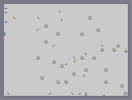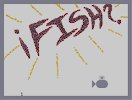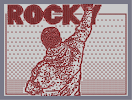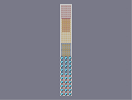Bunch of Laser Drones Físh Rocky Balboa oims

Pages: (0)

### ummm

I think you miscounted Lucas